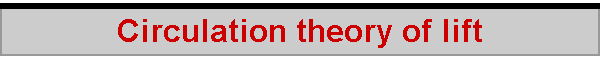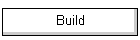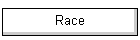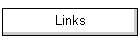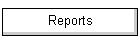The aerodynamicist's way of trying to understand the lift produced by a lifting surface is called the "circulation theory of lift".  This page considers a "simple" lifting surface of infinite length.  A wing of a fixed length is considered in the Lifting line theory of lift.  The circulation theory is similar to the momentum theory of lift, in that it too considers the reaction force on the wing (sail, fin) as a result of the wing "pushing" the air (water) downwards (sideways), but it looks a little more closely at the behaviour of the air flowing around the aerofoil.  This is how it works.First, we need to see what is meant by "circulation".  The idea is that, if a fluid is "circulating" around some object, the speed of some particle in the fluid is proportional to the distance to the centre of the circulation.  For a given amount of "circulation", the further away the circulating particles, the slower they move.  The amount of circulation is said to be the speed of the particles times the length of their circulation path.  Suppose their path is a circle.  The amount of circulation, called gamma, is given as 2 pi r, the circumference of the circle, times w, the speed of the circulating particles at some distance r from the centre. The idea is quite an interesting one.  Let us imagine we have gamma = 12.  This means that, at a distance of 1 from the centre, the particles are circulating with a speed of 12.  At a distance of 2, they circulate with a speed of 6.  At a distance 3, with a speed of 4, at a distance 4, with speed 3, and so on.  All of this is given when we say, the amount of circulation is 12. Here is a diagram of a foil moving through a fluid at a speed of V.  If we look at the rectangular path around this foil, which is "h" units high and "k" units long, and ask about the amount of circulation  we see, we can calculate it by multiplying the speed of the particles at each point around the rectangle and adding this up.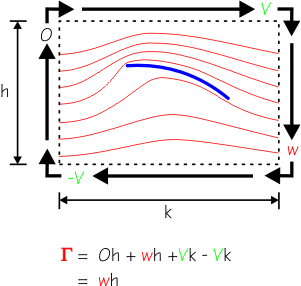Starting at the top of the rectangle, we see that the particles are travelling at a speed V, and they do so over the distance k;  the circulation here is "Vk". Down the right-hand side, the particles now show an average downwash speed w, along a distance h;  circulation here is "wh". Along the bottom, the speed of the particles is -V (relative to the direction of the path we are following), and they travel at this speed for the distance k, so circulation here is "-Vk".  Finally, up the left-hand side, we see that none of the particles are moving up or down, so their speed vertically is zero, 0.  The length of the path over which they travel at, er, zero speed is h, and so the circulation here is "0h".  Add up these regions of circulation, and we find that gamma, the overall amount of circulation is simply "wh".  The amount of circulation around the foil is given by the amount of downwash times the distance over which we consider the downwash to be operating. Let's now look at a wing (sail, fin) moving through the air (water) with velocity V.  Because of the wing's action, it causes some circulation in the air as the air passes by.  This is the same as saying that it causes some downwash.  We consider a portion of the wing, with a span of b, the wing chord to have length c, and the wing to cause some downwash with velocity w that acts within some arbitrary region of height h.  The circulation is gamma, equal to w times h, and our task is to deduce the lift of the wing.The lift force of the wing is given by considering that it has accelerated the air downwards, in a unit of time, giving it a downwash speed w.  This lift force is the mass of the air which has been deflected to an average downwash of w, times the downwash speed.  The mass of air so deflected in unit time is the density, rho, times its volume, V times b times h.  But we know that the amount of circulation around the wing, gamma, is equal to the downwash speed w, times the height of the region over which it operates, h.  This gives us the fundamental result of the circulation theory -- lift, the force acting at right angles to the air stream, equals the density of the air times the velocity of the air times the circulation, for a wing of unit span.A similar result holds when we consider the drag, which is the amount of force acting at right-angles to the average downwash.  Drag equals the density of the air times the downwash of the air times the circulation, for a wing of unit span.  Notice we say "wing of unit span".  This is because we consider the wing here to go on forever -- an infinite wing.  The circulation around a real wing, of a fixed span, is considered in the lifting line theory of lift. According to the circulation theory, we can now derive the lift coefficient from knowledge of the downwash speed.  Let us consider the circulation around the wing, from leading edge to trailing edge, and consider the circulation path as a circle whose diameter is the chord, c, of the wing.  On the one side of the equation, we calculate the lift using the lift coefficient in the usual way, and on the other side we calculate the lift using the theory of circulation.  We then obtain another fundamental result, that the lift coefficient is given by a constant times the ratio of the downwash speed to the air speed.  The higher the downwash relative to the speed of the air, the greater the lift coefficient.If we consider the case of a simple flat plate, and imagine that the plate deflects the air downwards, we can estimate that the angle of deflection is the angle of attack of the flat plate, alpha.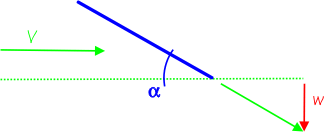And, we can estimate that the angle of deflection is approximately w divided by V for relatively small alpha.  In this case, the lift coefficient for a flat plate is 2 pi times the angle of attack.  When we convert from radians to degrees, this says that the lift coefficient increases by 0.11 for every degree of attack.  In fact, this result holds (in theory!) for more than a flat plate -- it holds for all lifting surfaces.Another view of the lift coefficient, based upon the amount of circulation, is given by a constant times the circulation divided by the air velocity and divided by the chord of the wing.  For other than rectangular planforms, we need to use the average chord.  This result is of less interest for us, though, since it is not often used, but it does point out something of interest.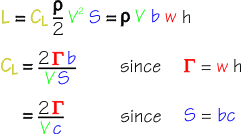The circulation, gamma, is a certain quantity at a given point along the wing, but can quite easily be a different amount at some other point along the wing.  For example, imagine that the wing, or sail, has considerable wash-out (or twist), such that the lift being generated towards the wing tip or sail head is considerably reduced.  This is the same as saying that the circulation in that region of the wing is considerably reduced.  Some pretty advanced mathematical techniques are need to calculate total circulation around a wing if the circulation around sections of the wing is different. This formula says that the wing lift coefficient, and hence the lift force, is a function of the circulation per unit chord, and this provides a hint of why the elliptical planform works -- the tapering of the chord towards the tip is exactly what is needed to "taper off" the circulation as well in order to keep lift constant along the wing. 2005-12-18